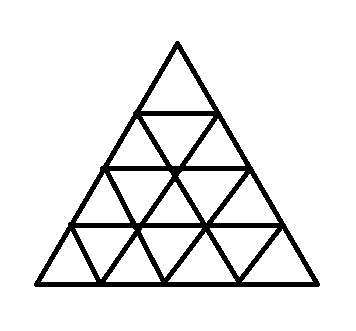# Python Program to Solve Triangular Matchstick Number

In the previous article, we have discussed Python Program to Compute Euclidean Distance
Given the number of sub triangles in the base layer and the task is to find the total number of sticks required to form the entire triangle.

Triangular Matchstick Number in Python :

Find the total number of matchsticks required to build the overall triangle until there is a single triangle at the top, given the number of sub-triangles in the base layer of the bigger triangle. For more information, please see the image below.Consider each triangle in the above image to be made of three matchsticks. So the goal of the problem is to determine the total number of matchsticks required to construct the entire triangle.

The solution’s concept is as follows:

The picture clearly shows that the number of triangles at each level decreases by one starting at the bottom. If the bottom layer contains N sub triangles, the layer above it contains N – 1 sub triangles, and so on until the topmost layer contains 1 triangle. So the final triangle will have N + (N – 1) + (N – 2) +… + 1 sub triangles, which is also equal to (N* (N + 1)) / 2.

Multiply it by the number of sticks needed for each sub triangle to get the final answer. As a result, the final solution is as simple as 3 * (N * (N + 1)) / 2.

Therefore the Formula is – 3 * (N * (N + 1)) / 2.

Examples:

Example1:

Input:

Given no of sub triangle's in base layer = 4

Output:

The Total number of matchsticks needed to form a above given entire triangle =  30

Example 2:

Input:

Given no of sub triangle's in base layer = 8

Output:

The Total number of matchsticks needed to form a above given entire triangle =  108

## Program to Solve Triangular Matchstick Number

Below are the ways to Solve Triangular Matchstick Number

### Method #1: Using Mathematical Formula (Static input)

Approach:

• Give the number as static input and Store it in a variable.
• Calculate the total number of sub triangle’s required by using the above mathematical formula (N * (N + 1)) / 2) and store it in another variable.
• Multiply the above obtained total sub triangle’s with ‘3’ to get the total number of sticks required.
• Store it in another variable.
• Print the Total number of sticks required to form an above given entire triangle.
• The Exit of the program.

Below is the implementation:

# Give the number as static input and Store it in a variable.
no_bse_subtringls = 4
# Calculate the total number of sub triangle's required by using above mathematical formula
# (N * (N + 1)) / 2) and store it in another variable.
tot_subtringle = (no_bse_subtringls * (no_bse_subtringls + 1)) // 2
# Multiply the above obtained total sub triangle's with '3' to get the total number of
# sticks required and Store it in another variable.
tot_sticks = tot_subtringle * 3
# Print the Total number of sticks required to form a above given entire triangle.
print("The Total number of matchsticks needed to form a above given entire triangle = ", tot_sticks)


Output:

The Total number of matchsticks needed to form a above given entire triangle =  30

### Method #2: Using Mathematical Formula (User input)

Approach:

• Give the number as user input using the int(input()) function and store it in a variable.
• Calculate the total number of sub triangle’s required by using the above mathematical formula (N * (N + 1)) / 2) and store it in another variable.
• Multiply the above obtained total sub triangle’s with ‘3’ to get the total number of sticks required.
• Store it in another variable.
• Print the Total number of sticks required to form an above given entire triangle.
• The Exit of the program.

Below is the implementation:

# Give the number as user input using the int(input()) function and Store it in a variable.
no_bse_subtringls = int(input("Enter some random number =  "))
# Calculate the total number of sub triangle's required by using above mathematical formula
# (N * (N + 1)) / 2) and store it in another variable.
tot_subtringle = (no_bse_subtringls * (no_bse_subtringls + 1)) // 2
# Multiply the above obtained total sub triangle's with '3' to get the total number of
# sticks required and Store it in another variable.
tot_sticks = tot_subtringle * 3
# Print the Total number of sticks required to form a above given entire triangle.
print("The Total number of matchsticks needed to form a above given entire triangle = ", tot_sticks)


Output:

Enter some random number = 8
The Total number of matchsticks needed to form a above given entire triangle = 108

Explore more instances related to python concepts from Python Programming Examples Guide and get promoted from beginner to professional programmer level in Python Programming Language.